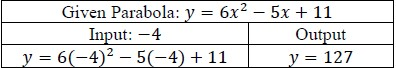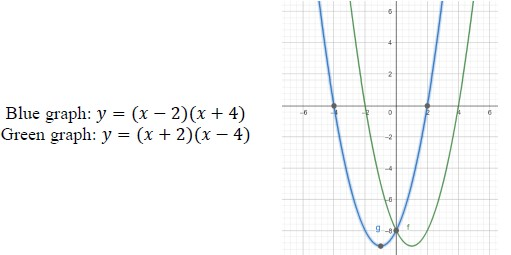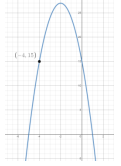# MA.912.AR.3.5Export Print
Given the x-intercepts and another point on the graph of a quadratic function, write the equation for the function.
General Information
Subject Area: Mathematics (B.E.S.T.)
Strand: Algebraic Reasoning
Status: State Board Approved

## Benchmark Instructional Guide

### Terms from the K-12 Glossary

• $x$-intercept
• $y$-intercept

### Vertical Alignment

Previous Benchmarks

Next Benchmarks

### Purpose and Instructional Strategies

In grade 8, students determined the slope in a linear relationship when given two points on the line. In Algebra I, students write the equation for a quadratic function when given the $x$-intercepts and another point on the graph. In later courses, students will write the equation for a quadratic function when given a vertex and another point on the graph.
• Instruction includes making connetions to various forms of quadratic equations to show their equivalency. Students should understand when one form might be more useful than other depending on the context.
• Standard Form Can be described by the equation $y$ = $a$$x$2+ $b$$x$ + $c$, where $a$, $b$ and $c$ are any rational number. This form can be useful when identifying the y-intercept.
• Factored Form Can be described by the equation $y$ = $a$($x$$r$1)($x$$r$2), where $r$1 and $r$2 are real numbers and the roots, or $x$-intercepts. This form can be useful when identifying the $x$-intercepts, or roots.
• Vertex Form Can be described by the equation $y$ = $a$($x$$h$)2  + $h$, where the point ($h$, $k$) is the vertex. This form can be useful when identifying the vertex.
• Instruction includes the use of $x$-$y$ notation and function notation.
• Instruction includes the use of graphing technology.

### Common Misconceptions or Errors

• Similar to their work with vertex form, students may have trouble with using the correct signs for $r$1 and  $r$2 in factored form. In these cases, show students a corresponding graph of their developed factored form functions to help them see the need for opposite signs in their factors.
• When rewriting their developed factored form functions into standard form, students may incorrectly multiply a by both factors. One remedy for this is to direct students to multiply their binomials first and then multiply the product by a.
• Students could see a problem that only presents one root and express confusion on how it applies to factored form. These cases provide an opportunity to discuss multiplicity of roots. Use graphing software to build the connection between the standard form of a quadratic function with a perfect square trinomial and the resulting parabola with only one root. Students should see that the root should be used for both $r$1 and $r$in factored form and confirm by converting their resulting function back to standard form.

### Strategies to Support Tiered Instruction

• Teacher provides instruction to show how different methods can be used to multiply binomials after substituting the roots into factored form. Then, ask students to recall methods to multiply binomials.
• Instruction includes converting a quadratic from vertex form to standard form. Instruct students to write out ($x$$h$)2 as ($x$$h$)($x$$h$). Remind students that different methods can be used to multiply binomials.
• Instruction includes an opportunity to discuss multiplicity of roots when converting from factored form to standard form. The teacher can use graphing software to build the connection between the standard form of a quadratic function with a perfect square trinomial and the resulting parabola with only one root. Students see that the root should be used for both $r$1 and $r$2 in factored form and confirm by converting their resulting function back to standard form.
• Teacher models how to solve for a by substituting the values of $x$ and $y$ from a point on the parabola.
• For students who need support evaluating functions, review the steps for evaluating a function given an input value by co-creating an anchor chart.• Teacher provides opportunities to use an online graphing tool to graph an equation with the $x$-intercepts to help students who may have used the wrong signs in their factors.
• For example, if the roots of a quadratic function are 2 and −4, the teacher can provide the graphs of the two functions (as shown below) to determine which equation corresponds to the roots.• A city fountain shoots jets of water that pass back and forth through a marble wall in its center. One jet of water begins 12 feet away from the wall and passes through a hole in the wall that is 12 feet high before landing 5 feet away on the other side. Write a quadratic function that represents the path the jet of water takes.

### Instructional Items

Instructional Item 1
• Write a quadratic function to represent the graph below.Instructional Item 2
• Write a quadratic function to represent a parabola with roots of 5 and −7 that passes through the point (−3, −128).

*The strategies, tasks and items included in the B1G-M are examples and should not be considered comprehensive.

## Related Courses

This benchmark is part of these courses.
1200310: Algebra 1 (Specifically in versions: 2014 - 2015, 2015 - 2022, 2022 and beyond (current))
1200320: Algebra 1 Honors (Specifically in versions: 2014 - 2015, 2015 - 2022, 2022 and beyond (current))
1200380: Algebra 1-B (Specifically in versions: 2014 - 2015, 2015 - 2022, 2022 and beyond (current))
1200400: Foundational Skills in Mathematics 9-12 (Specifically in versions: 2014 - 2015, 2015 - 2022, 2022 and beyond (current))
7912090: Access Algebra 1B (Specifically in versions: 2014 - 2015, 2015 - 2018, 2018 - 2019, 2019 - 2022, 2022 and beyond (current))
1200315: Algebra 1 for Credit Recovery (Specifically in versions: 2014 - 2015, 2015 - 2022, 2022 and beyond (current))
1200385: Algebra 1-B for Credit Recovery (Specifically in versions: 2014 - 2015, 2015 - 2022, 2022 and beyond (current))
7912075: Access Algebra 1 (Specifically in versions: 2014 - 2015, 2015 - 2018, 2018 - 2019, 2019 - 2022, 2022 and beyond (current))

## Related Access Points

Alternate version of this benchmark for students with significant cognitive disabilities.
MA.912.AR.3.AP.5:

Given the x-intercepts and another point on the graph of a quadratic function, select the equation for the function.

## Related Resources

Vetted resources educators can use to teach the concepts and skills in this benchmark.

## Student Resources

Vetted resources students can use to learn the concepts and skills in this benchmark.

## Parent Resources

Vetted resources caregivers can use to help students learn the concepts and skills in this benchmark.